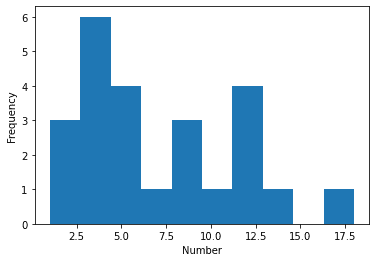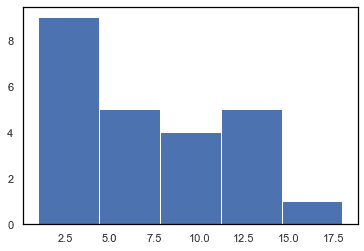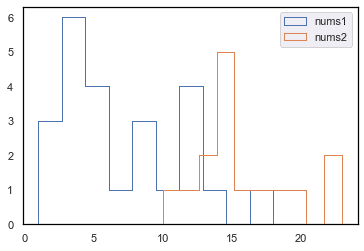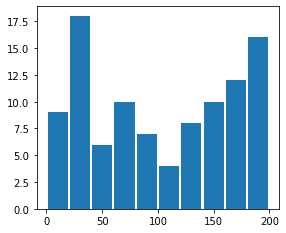search
Search
Publish
Guest 0reps
Thanks for the thanks!
close
account_circle
Profile
exit_to_app
Sign out
search
keyboard_voice
close
Searching Tips
Search for a recipe: "Creating a table in MySQL"
Search for an API documentation: "@append"
Search for code: "!dataframe"
Apply a tag filter: "#python"
Useful Shortcuts
/ to open search panel
Esc to close search panel
to navigate between search results
d to clear all current filters
Enter to expand content previewDoc SearchCode Search BetaSORRY NOTHING FOUND!
mic
Start speaking...Voice search is only supported in Safari and Chrome.
Shrink
Navigate to
A
A
share
thumb_up_alt
bookmark
arrow_backShareTwitterFacebook
thumb_up
0
thumb_down
0
chat_bubble_outline
0
auto_stories new
settings

# Drawing a histogram in Matplotlib

Programming
chevron_right
Python
chevron_right
Matplotlib
chevron_right
Cookbooks
chevron_right
Graphs Cookbook
schedule Mar 9, 2022
Last updated
local_offer PythonMatplotlib
Tags

We can use the `ax.hist(~)` method to draw a basic histogram in Matplotlib.

# Drawing a basic histogram

## Object Oriented Interface

To plot a histogram showing the distribution of some numbers:

``` nums = [1,1,2,3,3,3,3,3,4,5,6,6,6,7,8,8,9,10,12,12,12,12,14,18]fig,ax = plt.subplots()ax.hist(nums)ax.set_xlabel('Number')ax.set_ylabel('Frequency')plt.show() ```

This produces the following output:## Pyplot Interface

To plot the same histogram as above, but using Pyplot Interface:

``` nums = [1,1,2,3,3,3,3,3,4,5,6,6,6,7,8,8,9,10,12,12,12,12,14,18]_ = plt.hist(nums)_ = plt.xlabel('Number')_ = plt.ylabel('Frequency')plt.show() ```

It is good practice to assign the various plotting statements to a dummy variable `_` so that we do not display any unnecessary output.

# Setting the number of bins

By default the number of bins is 10, however, we can customize this using the `bins` argument:

``` nums = [1,1,2,3,3,3,3,3,4,5,6,6,6,7,8,8,9,10,12,12,12,12,14,18]fig,ax = plt.subplots()ax.hist(nums, bins=5)plt.show() ```

Now the output histogram only has 5 bins:# Multiple variables on the same histogram

We can plot multiple variables on the same histogram:

``` nums1 = [1,1,2,3,3,3,3,3,4,5,6,6,6,7,8,8,9,10,12,12,12,12,14,18]nums2= [10,12,13,13,14,14,15,15,15,16,17,18,20,22,23]fig,ax = plt.subplots()ax.hist(nums1, label="nums1", histtype="step")ax.hist(nums2, label="nums2", histtype="step")plt.legend()plt.show() ```

The `histtype="step"` means we only see the outlines of each histogram. This enables us to easily see the distribution for each variable when there is overlap between the two.# Visualizing the distribution of random numbers

We want to visualize the distribution of random numbers that we generate.

We generate 100 random integers between 0 (inclusive) and 200 (exclusive):

``` x = np.random.randint(low=0, high=200, size=100)x array([122, 76, 147, 160, 100, 173, 62, 21, 50, 150, 75, 19, 87, ... 83, 43, 184, 153, 148, 198, 88, 8, 196]) ```

We then draw a histogram showing the distribution of the data-points:

``` plt.hist(x, range=(0,200), bins=10, rwidth=0.9) ```

Here,

• `range` is a tuple indicating the lower and upper bound

• `bins` is number of bars you want

• `rwidth` is the width of each bin. If we set `rwidth=1`, then there will be no gap between the bins.

The output is as follows: